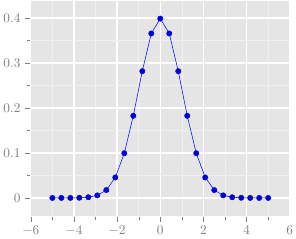# Plotting like ggplot2 with TikZ/Latex

I appreciate the graphics drawn by the ggplot2 package with R, results are nice and elegant. I decided to imitate the style of ggplot2 with TikZ. Here is a working example:

% Gauthier Vermandel 2014 \documentclass{standalone} \usepackage{pgfplotstable}     \definecolor{mygrey}{RGB}{229,229,229} \definecolor{mygrey2}{RGB}{127,127,127} \definecolor{mygrey3}{RGB}{240,240,240}     \pgfplotsset{ axis background/.style={fill=mygrey}, tick style=mygrey2, tick label style=mygrey2, grid=both, xtick pos=left, ytick pos=left, tick style={ major grid style={style=white,line width=1pt},minor grid style=mygrey3, tick align=outside, }, minor tick num=1, }     \begin{document} \begin{tikzpicture}   \begin{axis}[draw=white]   \addplot {1/sqrt(2*pi)*exp(-x^2/2)};   \end{axis}   \end{tikzpicture} \end{document}